Скачать презентацию Data Mining Clustering Cluster Analysis z What

d4cef0466ec5cda9035cf1386bda1b0c.ppt

• Количество слайдов: 45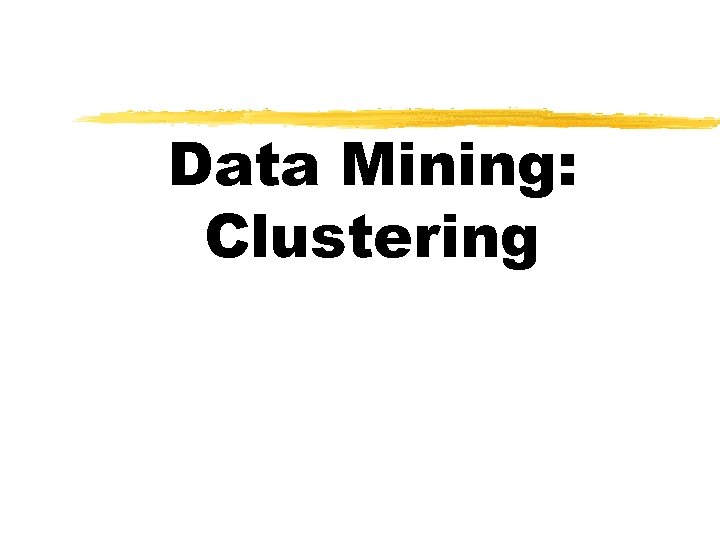Data Mining: Clustering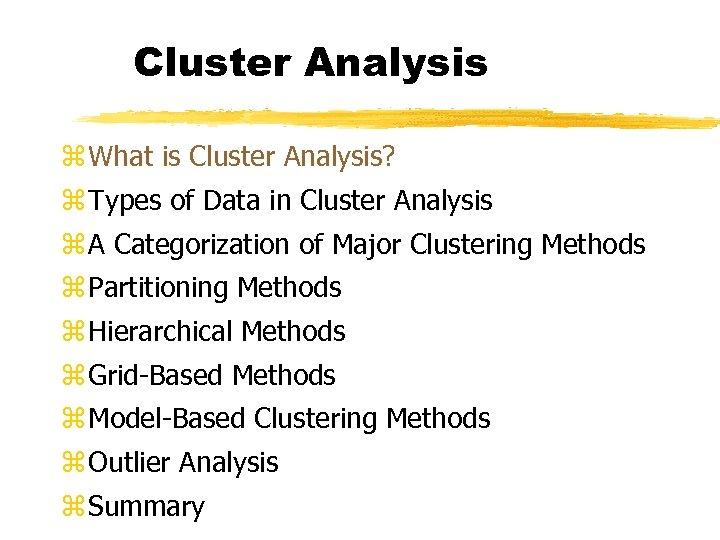Cluster Analysis z What is Cluster Analysis? z Types of Data in Cluster Analysis z A Categorization of Major Clustering Methods z Partitioning Methods z Hierarchical Methods z Grid-Based Methods z Model-Based Clustering Methods z Outlier Analysis z Summary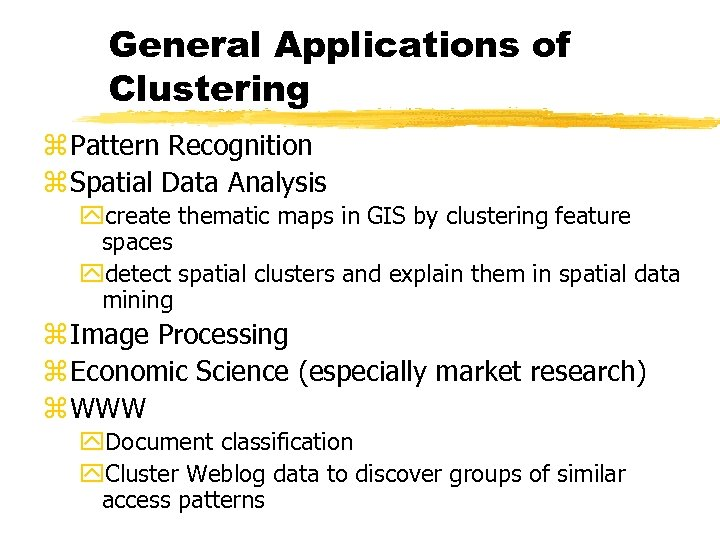General Applications of Clustering z Pattern Recognition z Spatial Data Analysis ycreate thematic maps in GIS by clustering feature spaces ydetect spatial clusters and explain them in spatial data mining z Image Processing z Economic Science (especially market research) z WWW y. Document classification y. Cluster Weblog data to discover groups of similar access patterns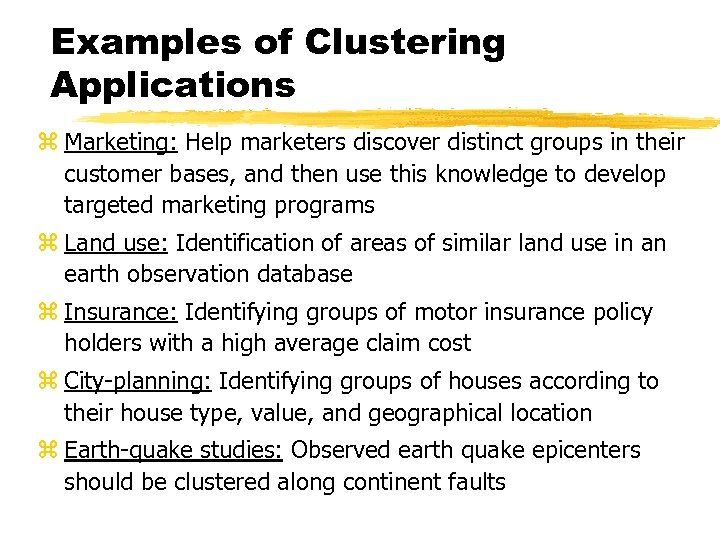Examples of Clustering Applications z Marketing: Help marketers discover distinct groups in their customer bases, and then use this knowledge to develop targeted marketing programs z Land use: Identification of areas of similar land use in an earth observation database z Insurance: Identifying groups of motor insurance policy holders with a high average claim cost z City-planning: Identifying groups of houses according to their house type, value, and geographical location z Earth-quake studies: Observed earth quake epicenters should be clustered along continent faults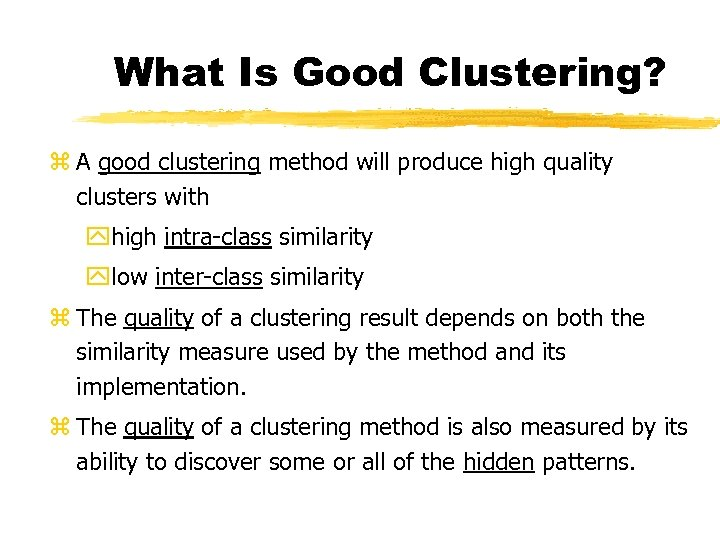What Is Good Clustering? z A good clustering method will produce high quality clusters with yhigh intra-class similarity ylow inter-class similarity z The quality of a clustering result depends on both the similarity measure used by the method and its implementation. z The quality of a clustering method is also measured by its ability to discover some or all of the hidden patterns.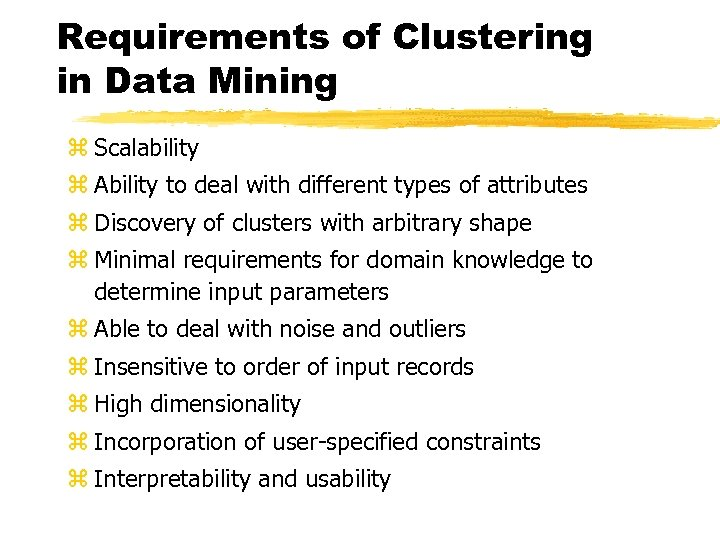Requirements of Clustering in Data Mining z Scalability z Ability to deal with different types of attributes z Discovery of clusters with arbitrary shape z Minimal requirements for domain knowledge to determine input parameters z Able to deal with noise and outliers z Insensitive to order of input records z High dimensionality z Incorporation of user-specified constraints z Interpretability and usability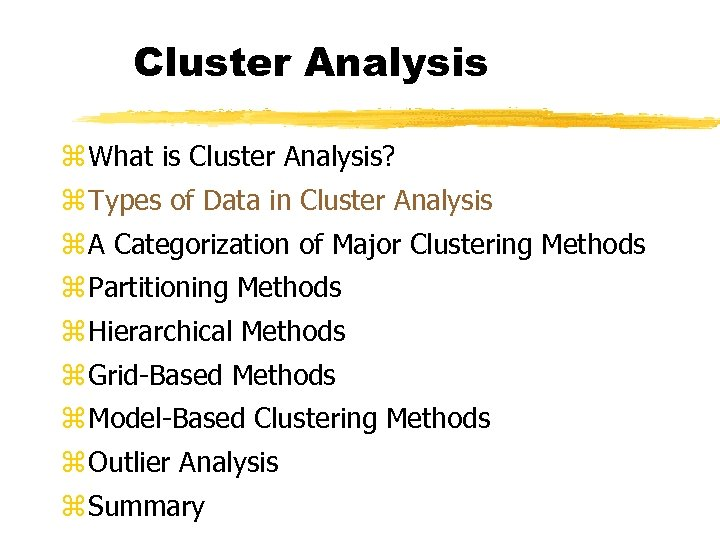Cluster Analysis z What is Cluster Analysis? z Types of Data in Cluster Analysis z A Categorization of Major Clustering Methods z Partitioning Methods z Hierarchical Methods z Grid-Based Methods z Model-Based Clustering Methods z Outlier Analysis z Summary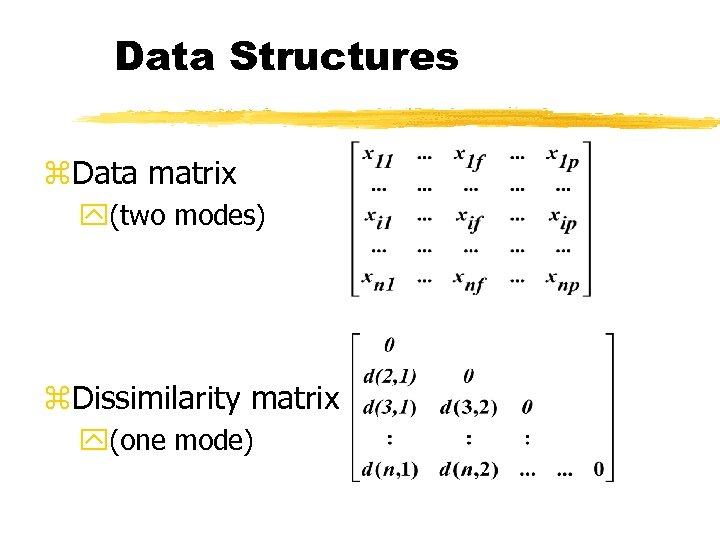Data Structures z. Data matrix y(two modes) z. Dissimilarity matrix y(one mode)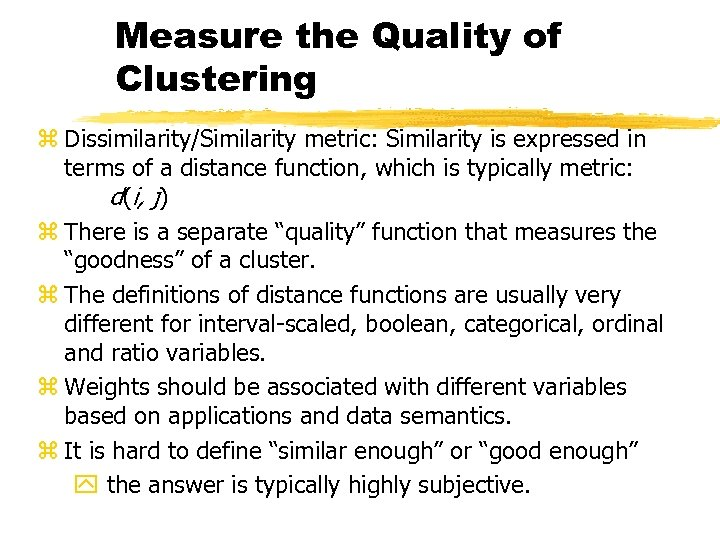Measure the Quality of Clustering z Dissimilarity/Similarity metric: Similarity is expressed in terms of a distance function, which is typically metric: d(i, j) z There is a separate “quality” function that measures the “goodness” of a cluster. z The definitions of distance functions are usually very different for interval-scaled, boolean, categorical, ordinal and ratio variables. z Weights should be associated with different variables based on applications and data semantics. z It is hard to define “similar enough” or “good enough” y the answer is typically highly subjective.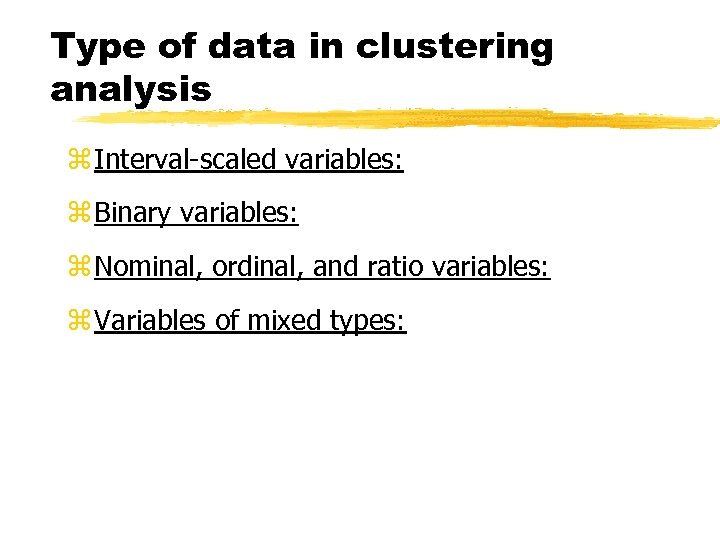Type of data in clustering analysis z Interval-scaled variables: z Binary variables: z Nominal, ordinal, and ratio variables: z Variables of mixed types: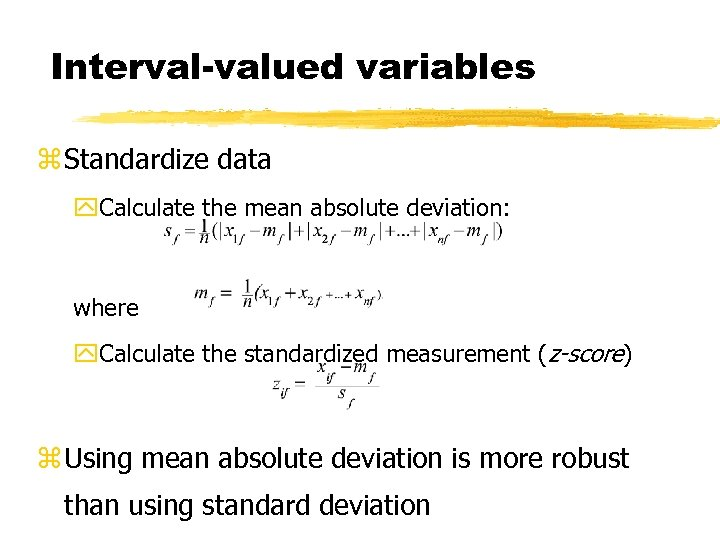Interval-valued variables z Standardize data y. Calculate the mean absolute deviation: where y. Calculate the standardized measurement (z-score) z Using mean absolute deviation is more robust than using standard deviation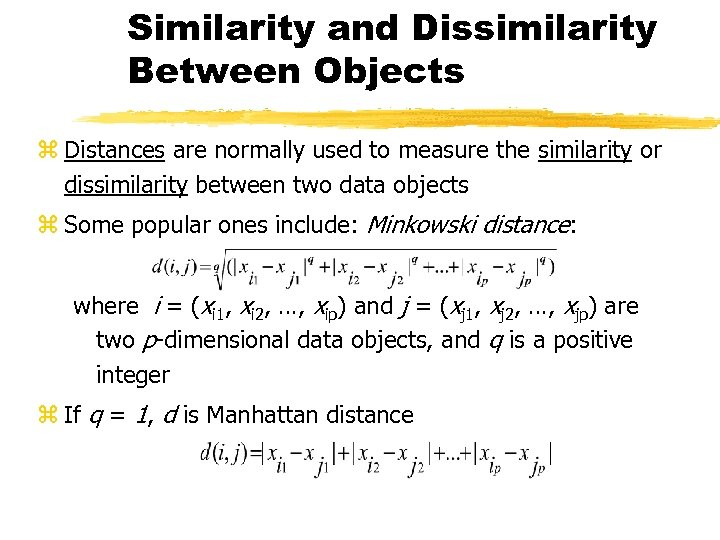Similarity and Dissimilarity Between Objects z Distances are normally used to measure the similarity or dissimilarity between two data objects z Some popular ones include: Minkowski distance: where i = (xi 1, xi 2, …, xip) and j = (xj 1, xj 2, …, xjp) are two p-dimensional data objects, and q is a positive integer z If q = 1, d is Manhattan distance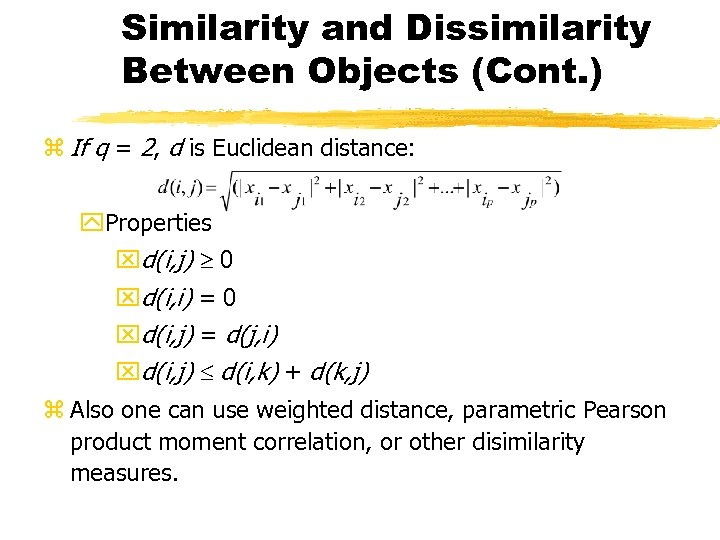Similarity and Dissimilarity Between Objects (Cont. ) z If q = 2, d is Euclidean distance: y. Properties xd(i, j) 0 xd(i, i) = 0 xd(i, j) = d(j, i) xd(i, j) d(i, k) + d(k, j) z Also one can use weighted distance, parametric Pearson product moment correlation, or other disimilarity measures.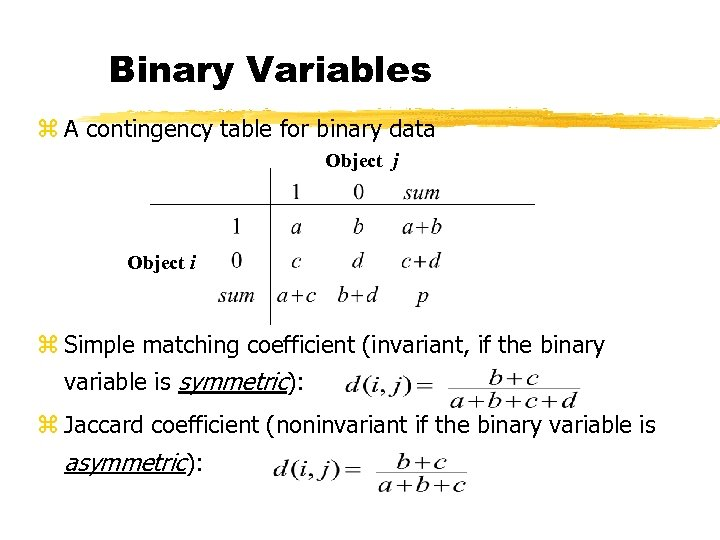Binary Variables z A contingency table for binary data Object j Object i z Simple matching coefficient (invariant, if the binary variable is symmetric): z Jaccard coefficient (noninvariant if the binary variable is asymmetric):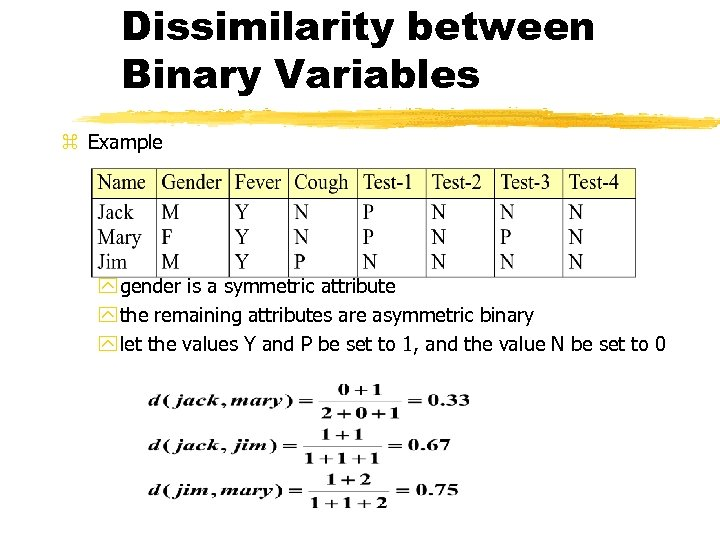Dissimilarity between Binary Variables z Example y gender is a symmetric attribute y the remaining attributes are asymmetric binary y let the values Y and P be set to 1, and the value N be set to 0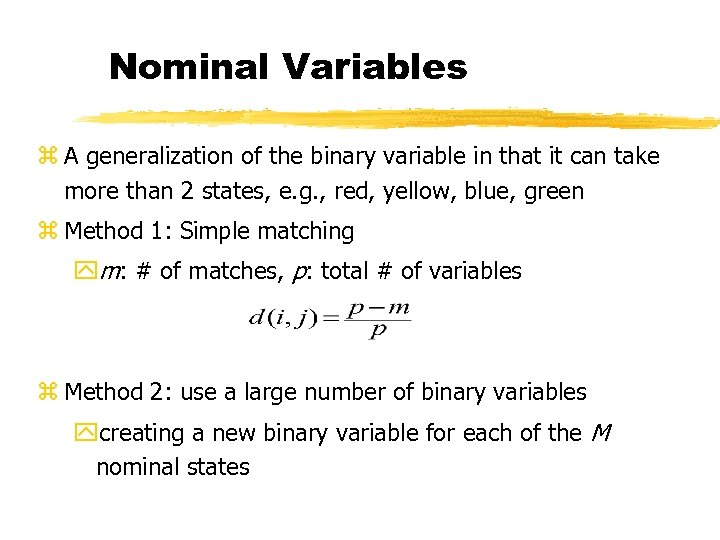Nominal Variables z A generalization of the binary variable in that it can take more than 2 states, e. g. , red, yellow, blue, green z Method 1: Simple matching ym: # of matches, p: total # of variables z Method 2: use a large number of binary variables ycreating a new binary variable for each of the M nominal states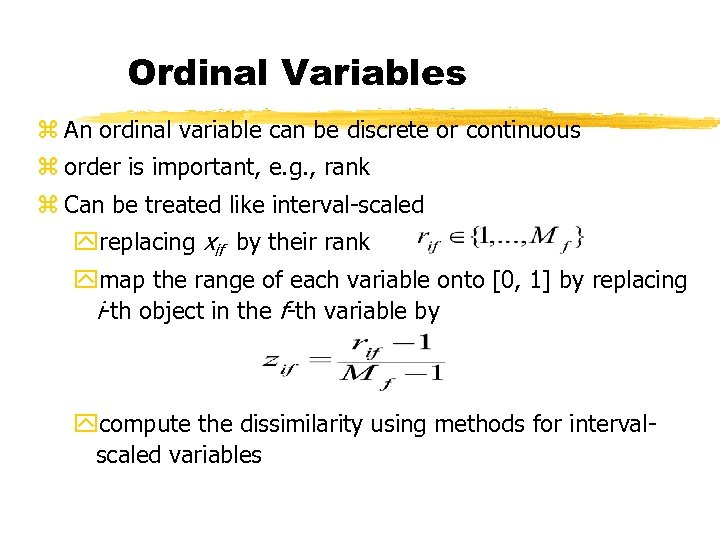Ordinal Variables z An ordinal variable can be discrete or continuous z order is important, e. g. , rank z Can be treated like interval-scaled yreplacing xif by their rank ymap the range of each variable onto [0, 1] by replacing i-th object in the f-th variable by ycompute the dissimilarity using methods for intervalscaled variables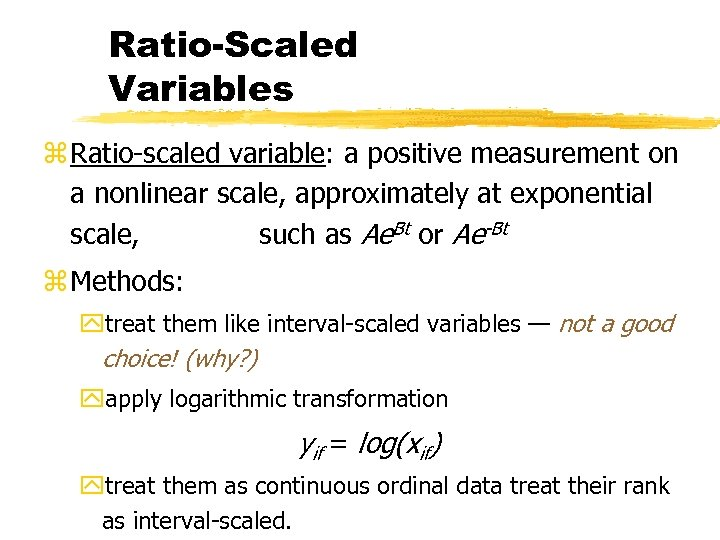Ratio-Scaled Variables z Ratio-scaled variable: a positive measurement on a nonlinear scale, approximately at exponential scale, such as Ae. Bt or Ae-Bt z Methods: ytreat them like interval-scaled variables — not a good choice! (why? ) yapply logarithmic transformation yif = log(xif) ytreat them as continuous ordinal data treat their rank as interval-scaled.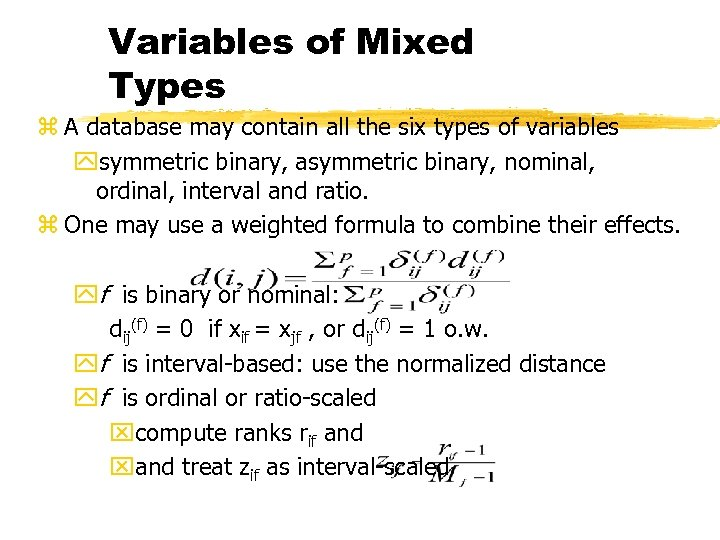Variables of Mixed Types z A database may contain all the six types of variables ysymmetric binary, asymmetric binary, nominal, ordinal, interval and ratio. z One may use a weighted formula to combine their effects. yf is binary or nominal: dij(f) = 0 if xif = xjf , or dij(f) = 1 o. w. yf is interval-based: use the normalized distance yf is ordinal or ratio-scaled xcompute ranks rif and xand treat zif as interval-scaled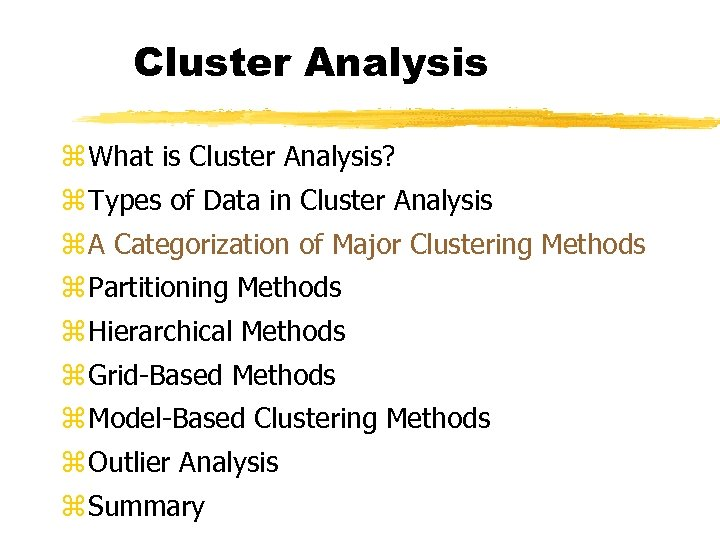Cluster Analysis z What is Cluster Analysis? z Types of Data in Cluster Analysis z A Categorization of Major Clustering Methods z Partitioning Methods z Hierarchical Methods z Grid-Based Methods z Model-Based Clustering Methods z Outlier Analysis z Summary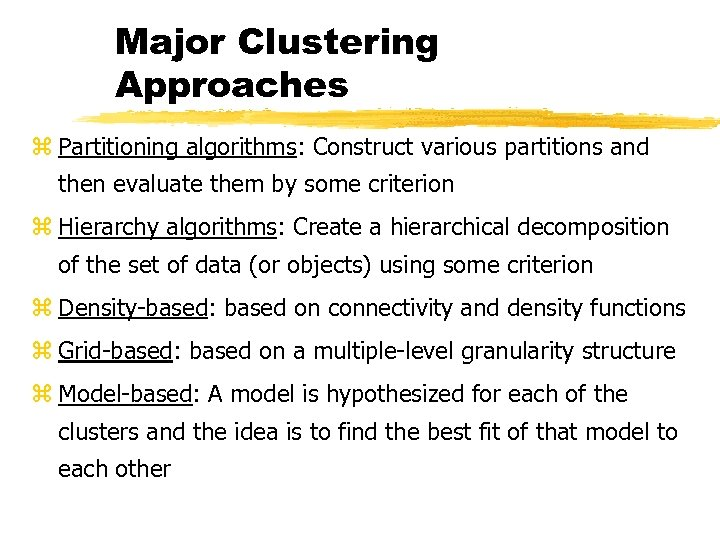Major Clustering Approaches z Partitioning algorithms: Construct various partitions and then evaluate them by some criterion z Hierarchy algorithms: Create a hierarchical decomposition of the set of data (or objects) using some criterion z Density-based: based on connectivity and density functions z Grid-based: based on a multiple-level granularity structure z Model-based: A model is hypothesized for each of the clusters and the idea is to find the best fit of that model to each other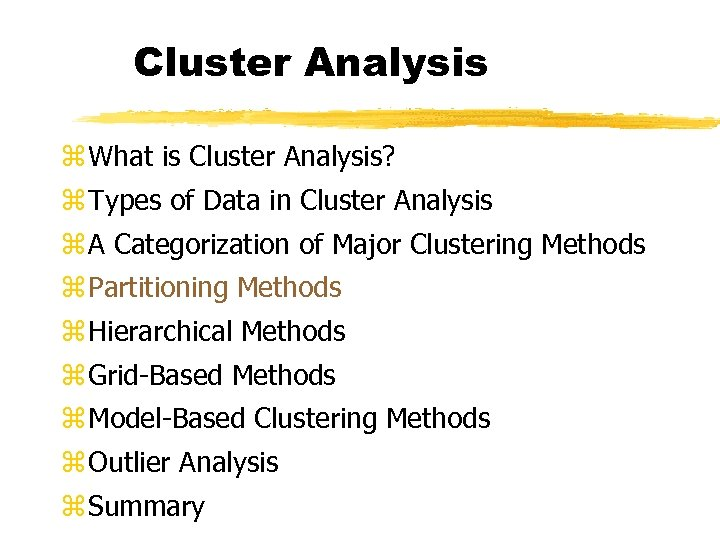Cluster Analysis z What is Cluster Analysis? z Types of Data in Cluster Analysis z A Categorization of Major Clustering Methods z Partitioning Methods z Hierarchical Methods z Grid-Based Methods z Model-Based Clustering Methods z Outlier Analysis z Summary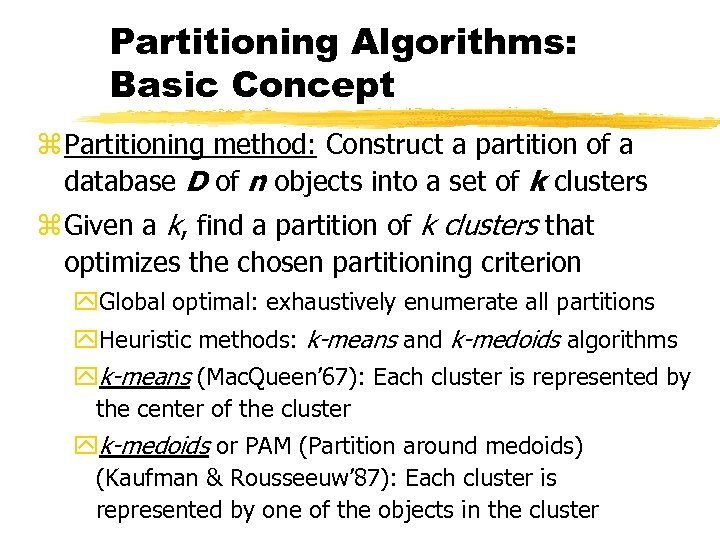Partitioning Algorithms: Basic Concept z Partitioning method: Construct a partition of a database D of n objects into a set of k clusters z Given a k, find a partition of k clusters that optimizes the chosen partitioning criterion y. Global optimal: exhaustively enumerate all partitions y. Heuristic methods: k-means and k-medoids algorithms yk-means (Mac. Queen’ 67): Each cluster is represented by the center of the cluster yk-medoids or PAM (Partition around medoids) (Kaufman & Rousseeuw’ 87): Each cluster is represented by one of the objects in the cluster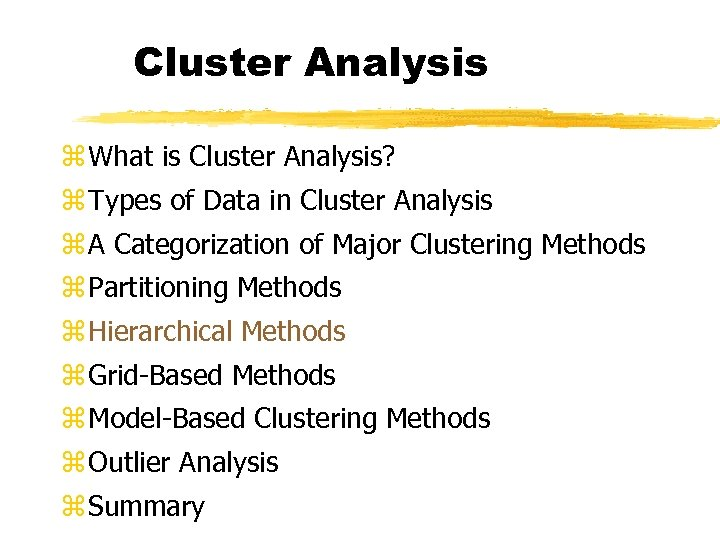Cluster Analysis z What is Cluster Analysis? z Types of Data in Cluster Analysis z A Categorization of Major Clustering Methods z Partitioning Methods z Hierarchical Methods z Grid-Based Methods z Model-Based Clustering Methods z Outlier Analysis z Summary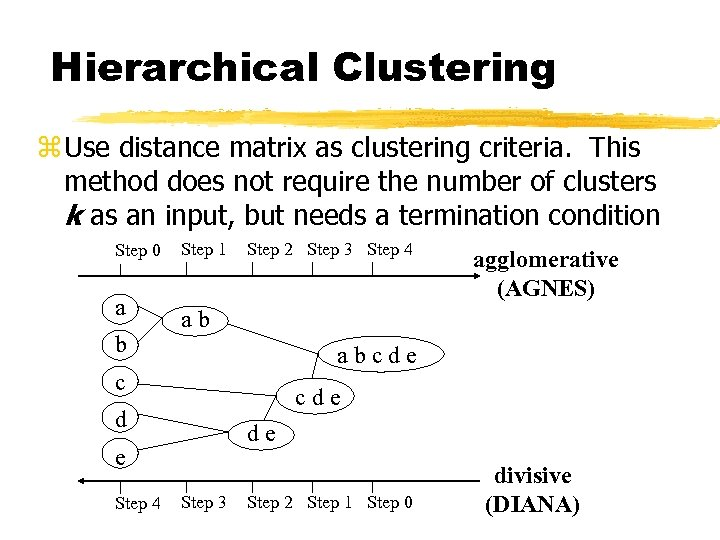Hierarchical Clustering z Use distance matrix as clustering criteria. This method does not require the number of clusters k as an input, but needs a termination condition Step 0 a b Step 1 Step 2 Step 3 Step 4 ab abcde c cde d de e Step 4 agglomerative (AGNES) Step 3 Step 2 Step 1 Step 0 divisive (DIANA)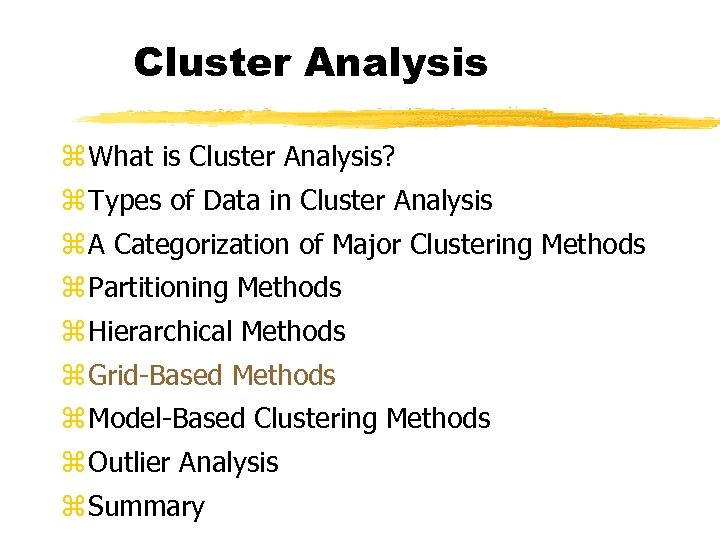Cluster Analysis z What is Cluster Analysis? z Types of Data in Cluster Analysis z A Categorization of Major Clustering Methods z Partitioning Methods z Hierarchical Methods z Grid-Based Methods z Model-Based Clustering Methods z Outlier Analysis z Summary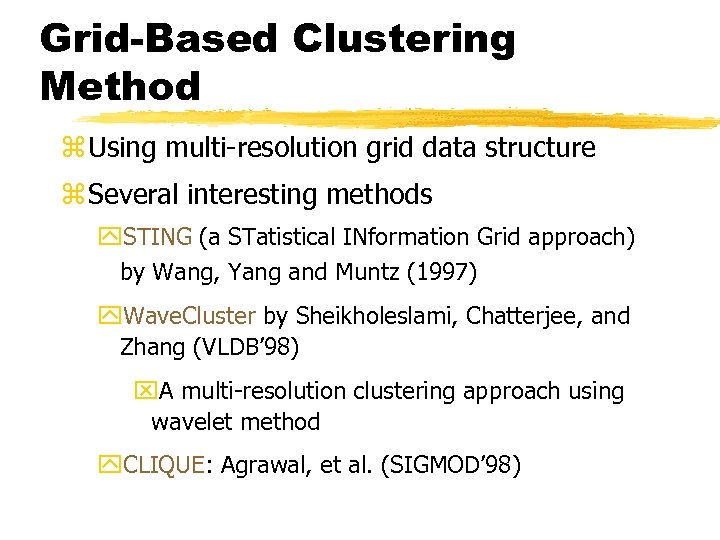Grid-Based Clustering Method z Using multi-resolution grid data structure z Several interesting methods y. STING (a STatistical INformation Grid approach) by Wang, Yang and Muntz (1997) y. Wave. Cluster by Sheikholeslami, Chatterjee, and Zhang (VLDB’ 98) x. A multi-resolution clustering approach using wavelet method y. CLIQUE: Agrawal, et al. (SIGMOD’ 98)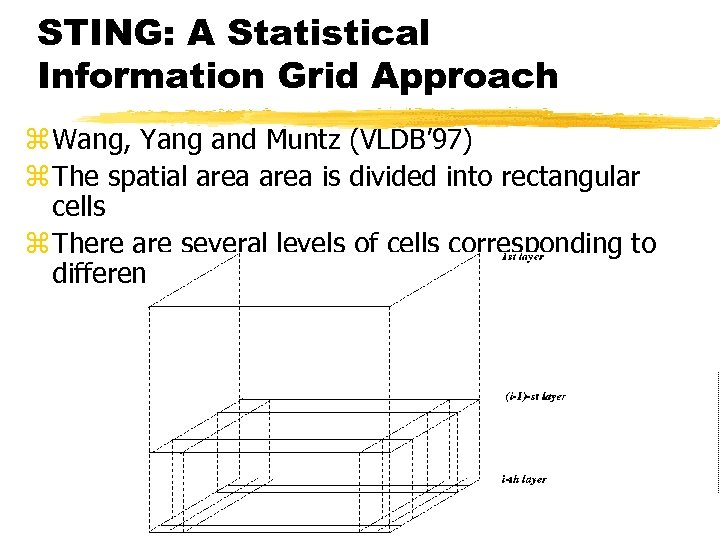STING: A Statistical Information Grid Approach z Wang, Yang and Muntz (VLDB’ 97) z The spatial area is divided into rectangular cells z There are several levels of cells corresponding to different levels of resolution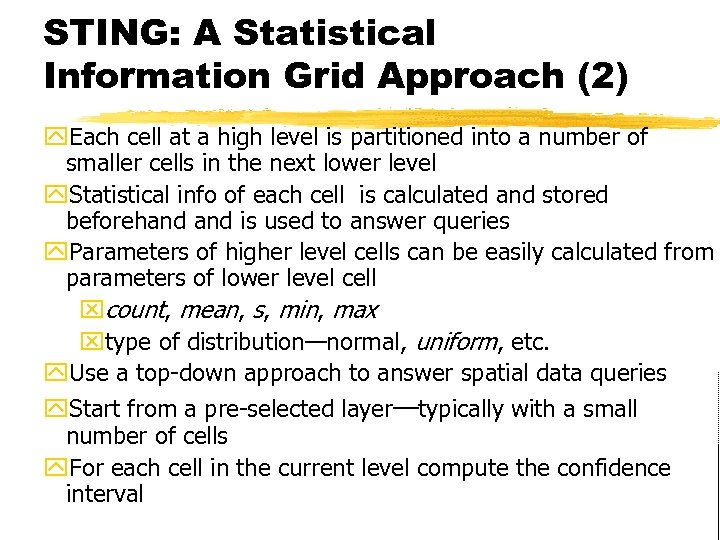STING: A Statistical Information Grid Approach (2) y. Each cell at a high level is partitioned into a number of smaller cells in the next lower level y. Statistical info of each cell is calculated and stored beforehand is used to answer queries y. Parameters of higher level cells can be easily calculated from parameters of lower level cell xcount, mean, s, min, max xtype of distribution—normal, uniform, etc. y. Use a top-down approach to answer spatial data queries y. Start from a pre-selected layer—typically with a small number of cells y. For each cell in the current level compute the confidence interval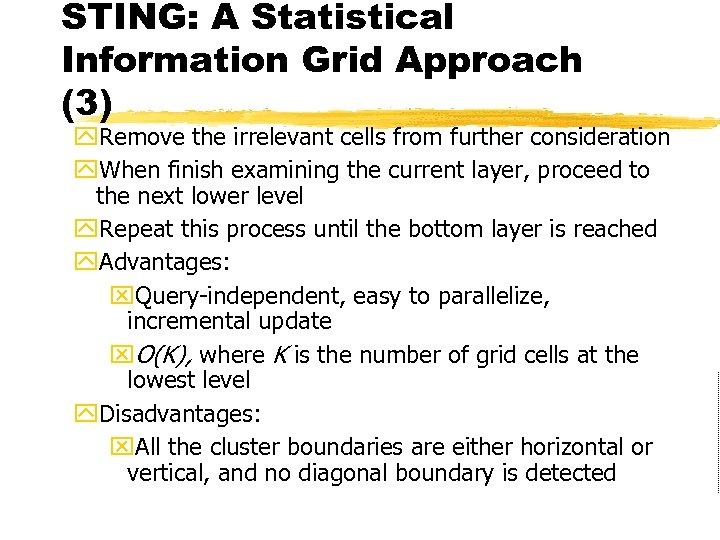STING: A Statistical Information Grid Approach (3) y. Remove the irrelevant cells from further consideration y. When finish examining the current layer, proceed to the next lower level y. Repeat this process until the bottom layer is reached y. Advantages: x. Query-independent, easy to parallelize, incremental update x. O(K), where K is the number of grid cells at the lowest level y. Disadvantages: x. All the cluster boundaries are either horizontal or vertical, and no diagonal boundary is detected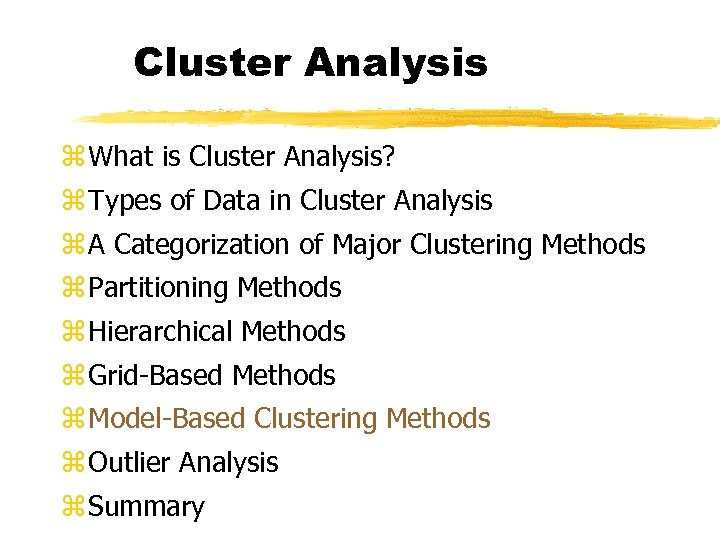Cluster Analysis z What is Cluster Analysis? z Types of Data in Cluster Analysis z A Categorization of Major Clustering Methods z Partitioning Methods z Hierarchical Methods z Grid-Based Methods z Model-Based Clustering Methods z Outlier Analysis z SummaryModel-Based Clustering Methods z Attempt to optimize the fit between the data and some mathematical model z Statistical and AI approach y. Conceptual clustering x. A form of clustering in machine learning x. Produces a classification scheme for a set of unlabeled objects x. Finds characteristic description for each concept (class) y. COBWEB (Fisher’ 87) x. A popular a simple method of incremental conceptual learning x. Creates a hierarchical clustering in the form of a classification tree x. Each node refers to a concept and contains a probabilistic description of that concept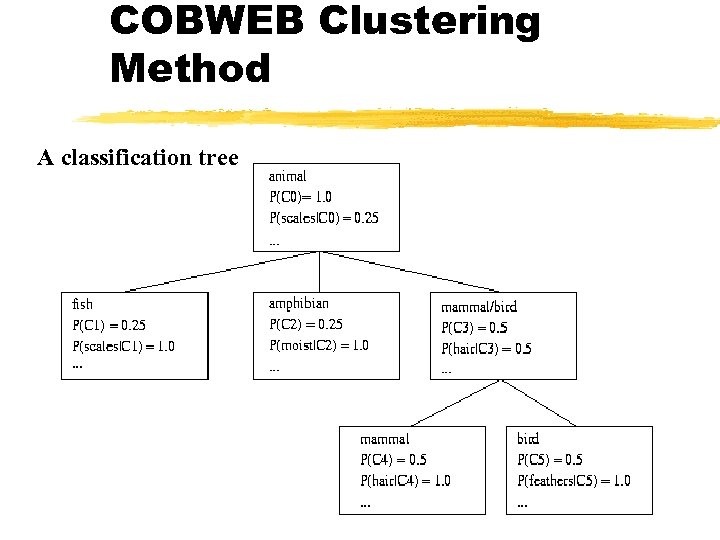COBWEB Clustering Method A classification tree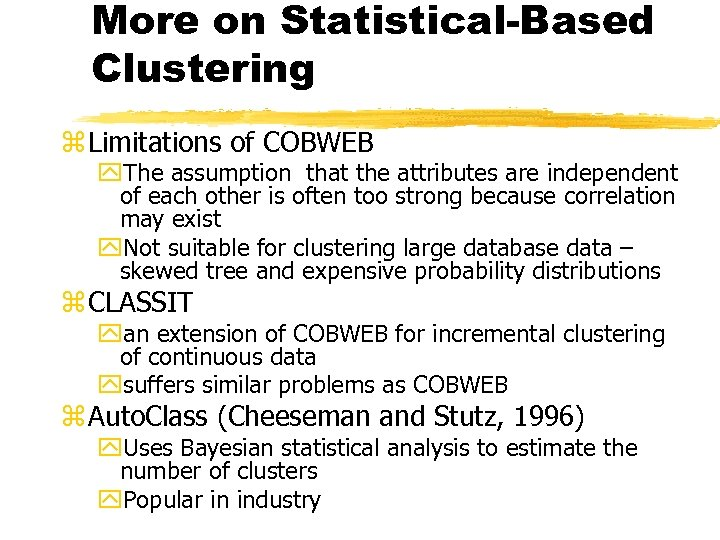More on Statistical-Based Clustering z Limitations of COBWEB y. The assumption that the attributes are independent of each other is often too strong because correlation may exist y. Not suitable for clustering large database data – skewed tree and expensive probability distributions z CLASSIT yan extension of COBWEB for incremental clustering of continuous data ysuffers similar problems as COBWEB z Auto. Class (Cheeseman and Stutz, 1996) y. Uses Bayesian statistical analysis to estimate the number of clusters y. Popular in industry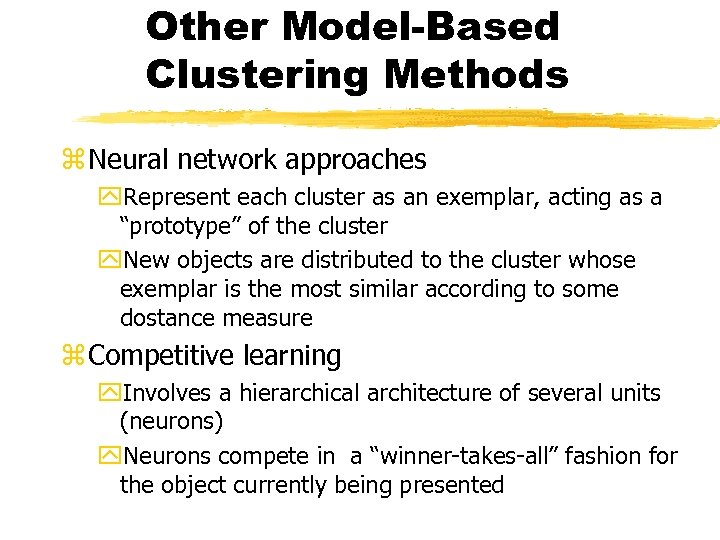Other Model-Based Clustering Methods z Neural network approaches y. Represent each cluster as an exemplar, acting as a “prototype” of the cluster y. New objects are distributed to the cluster whose exemplar is the most similar according to some dostance measure z Competitive learning y. Involves a hierarchical architecture of several units (neurons) y. Neurons compete in a “winner-takes-all” fashion for the object currently being presented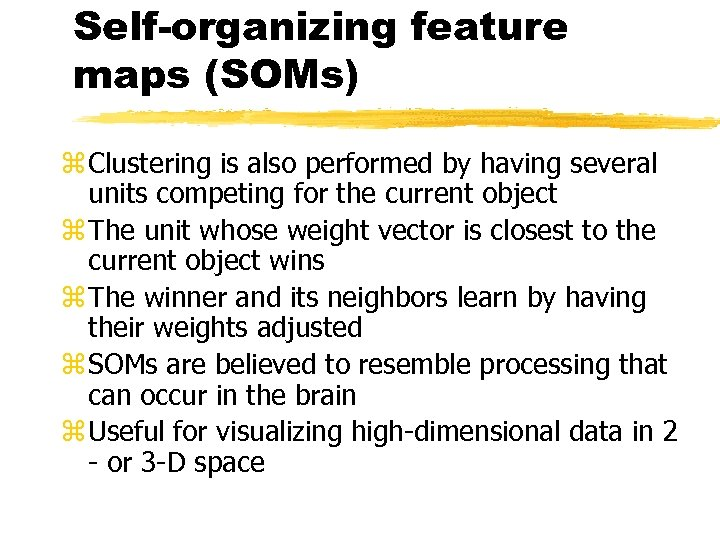Self-organizing feature maps (SOMs) z Clustering is also performed by having several units competing for the current object z The unit whose weight vector is closest to the current object wins z The winner and its neighbors learn by having their weights adjusted z SOMs are believed to resemble processing that can occur in the brain z Useful for visualizing high-dimensional data in 2 - or 3 -D space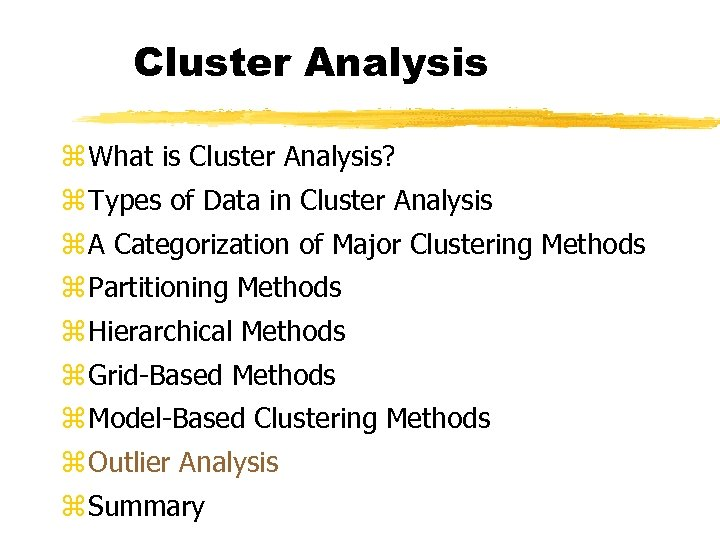Cluster Analysis z What is Cluster Analysis? z Types of Data in Cluster Analysis z A Categorization of Major Clustering Methods z Partitioning Methods z Hierarchical Methods z Grid-Based Methods z Model-Based Clustering Methods z Outlier Analysis z Summary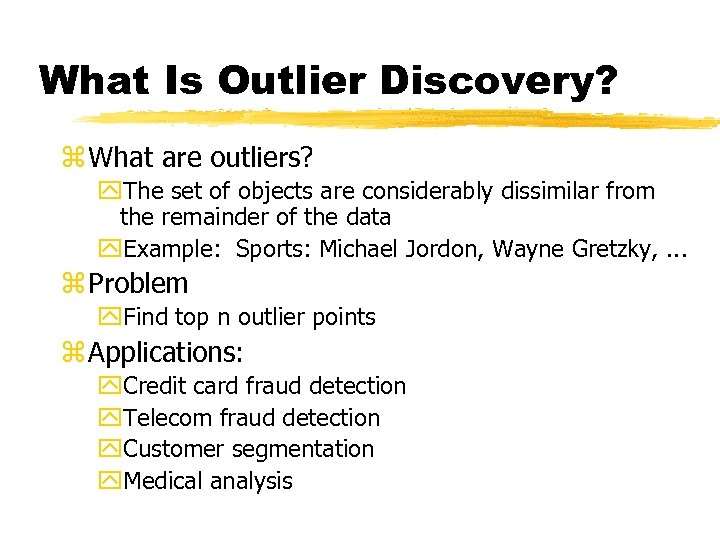What Is Outlier Discovery? z What are outliers? y. The set of objects are considerably dissimilar from the remainder of the data y. Example: Sports: Michael Jordon, Wayne Gretzky, . . . z Problem y. Find top n outlier points z Applications: y. Credit card fraud detection y. Telecom fraud detection y. Customer segmentation y. Medical analysis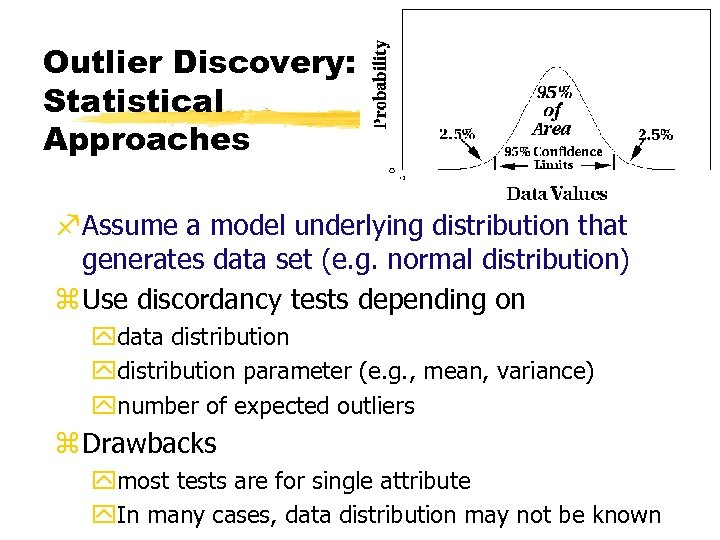Outlier Discovery: Statistical Approaches f. Assume a model underlying distribution that generates data set (e. g. normal distribution) z Use discordancy tests depending on ydata distribution ydistribution parameter (e. g. , mean, variance) ynumber of expected outliers z Drawbacks ymost tests are for single attribute y. In many cases, data distribution may not be known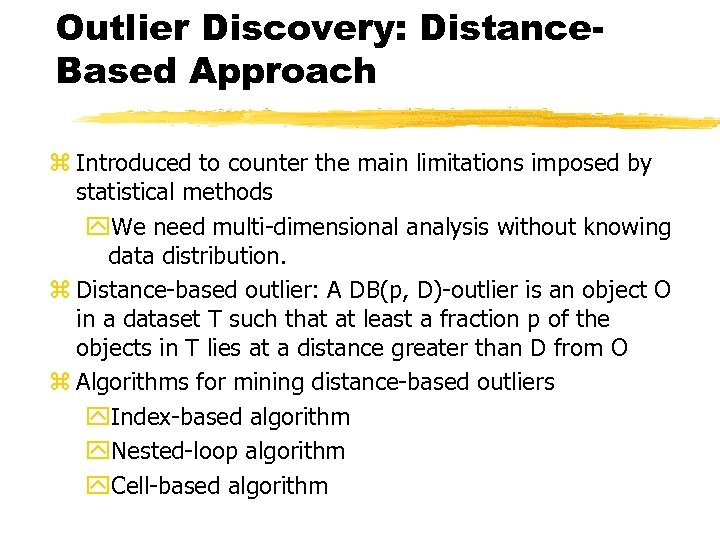Outlier Discovery: Distance. Based Approach z Introduced to counter the main limitations imposed by statistical methods y. We need multi-dimensional analysis without knowing data distribution. z Distance-based outlier: A DB(p, D)-outlier is an object O in a dataset T such that at least a fraction p of the objects in T lies at a distance greater than D from O z Algorithms for mining distance-based outliers y. Index-based algorithm y. Nested-loop algorithm y. Cell-based algorithm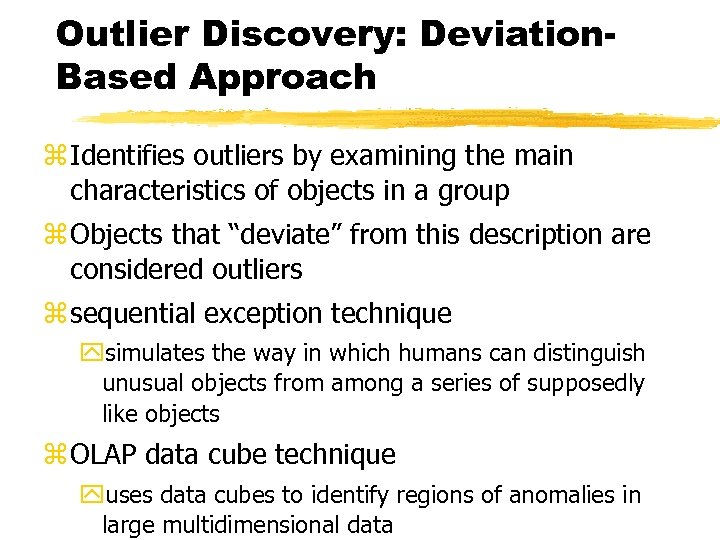Outlier Discovery: Deviation. Based Approach z Identifies outliers by examining the main characteristics of objects in a group z Objects that “deviate” from this description are considered outliers z sequential exception technique ysimulates the way in which humans can distinguish unusual objects from among a series of supposedly like objects z OLAP data cube technique yuses data cubes to identify regions of anomalies in large multidimensional data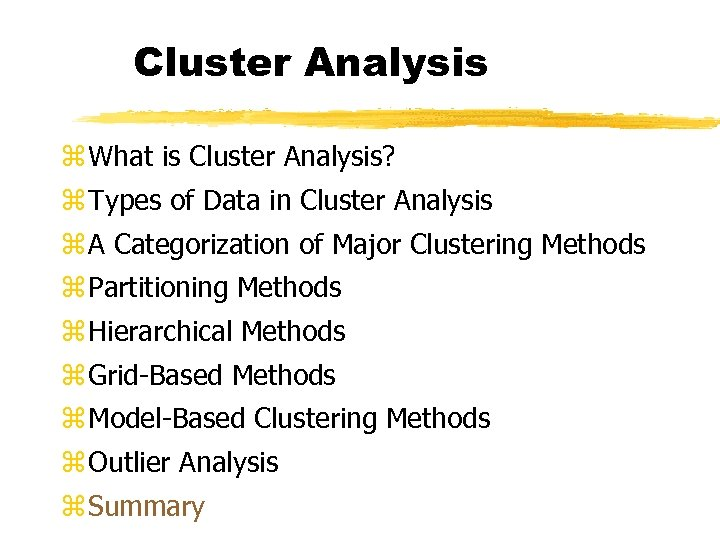Cluster Analysis z What is Cluster Analysis? z Types of Data in Cluster Analysis z A Categorization of Major Clustering Methods z Partitioning Methods z Hierarchical Methods z Grid-Based Methods z Model-Based Clustering Methods z Outlier Analysis z Summary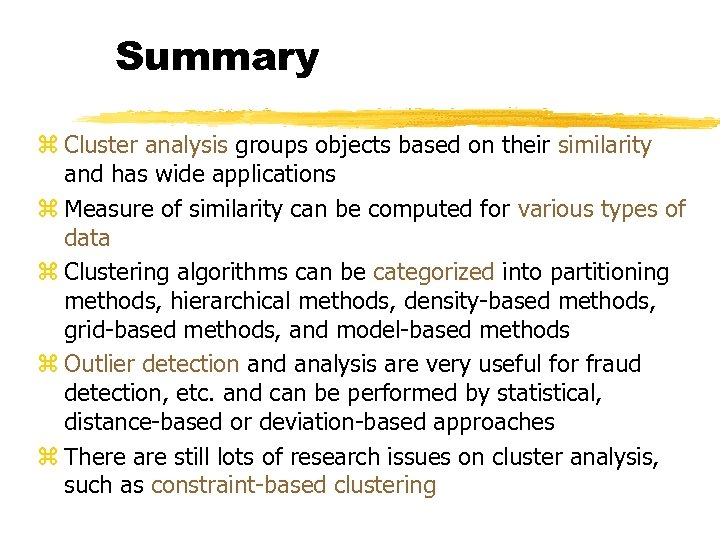Summary z Cluster analysis groups objects based on their similarity and has wide applications z Measure of similarity can be computed for various types of data z Clustering algorithms can be categorized into partitioning methods, hierarchical methods, density-based methods, grid-based methods, and model-based methods z Outlier detection and analysis are very useful for fraud detection, etc. and can be performed by statistical, distance-based or deviation-based approaches z There are still lots of research issues on cluster analysis, such as constraint-based clustering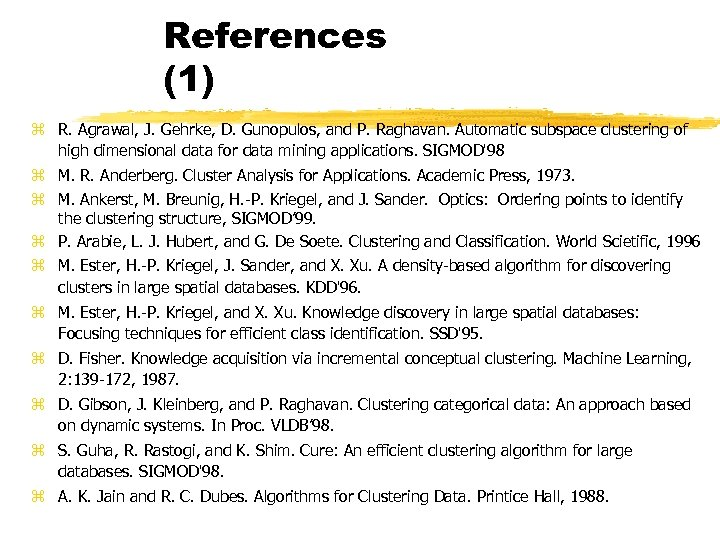References (1) z R. Agrawal, J. Gehrke, D. Gunopulos, and P. Raghavan. Automatic subspace clustering of high dimensional data for data mining applications. SIGMOD'98 z M. R. Anderberg. Cluster Analysis for Applications. Academic Press, 1973. z M. Ankerst, M. Breunig, H. -P. Kriegel, and J. Sander. Optics: Ordering points to identify the clustering structure, SIGMOD’ 99. z P. Arabie, L. J. Hubert, and G. De Soete. Clustering and Classification. World Scietific, 1996 z M. Ester, H. -P. Kriegel, J. Sander, and X. Xu. A density-based algorithm for discovering clusters in large spatial databases. KDD'96. z M. Ester, H. -P. Kriegel, and X. Xu. Knowledge discovery in large spatial databases: Focusing techniques for efficient class identification. SSD'95. z D. Fisher. Knowledge acquisition via incremental conceptual clustering. Machine Learning, 2: 139 -172, 1987. z D. Gibson, J. Kleinberg, and P. Raghavan. Clustering categorical data: An approach based on dynamic systems. In Proc. VLDB’ 98. z S. Guha, R. Rastogi, and K. Shim. Cure: An efficient clustering algorithm for large databases. SIGMOD'98. z A. K. Jain and R. C. Dubes. Algorithms for Clustering Data. Printice Hall, 1988.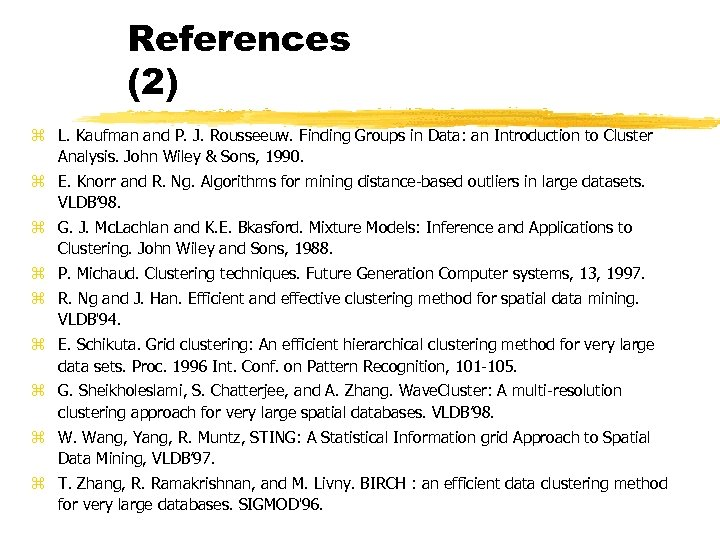References (2) z L. Kaufman and P. J. Rousseeuw. Finding Groups in Data: an Introduction to Cluster Analysis. John Wiley & Sons, 1990. z E. Knorr and R. Ng. Algorithms for mining distance-based outliers in large datasets. VLDB’ 98. z G. J. Mc. Lachlan and K. E. Bkasford. Mixture Models: Inference and Applications to Clustering. John Wiley and Sons, 1988. z P. Michaud. Clustering techniques. Future Generation Computer systems, 13, 1997. z R. Ng and J. Han. Efficient and effective clustering method for spatial data mining. VLDB'94. z E. Schikuta. Grid clustering: An efficient hierarchical clustering method for very large data sets. Proc. 1996 Int. Conf. on Pattern Recognition, 101 -105. z G. Sheikholeslami, S. Chatterjee, and A. Zhang. Wave. Cluster: A multi-resolution clustering approach for very large spatial databases. VLDB’ 98. z W. Wang, Yang, R. Muntz, STING: A Statistical Information grid Approach to Spatial Data Mining, VLDB’ 97. z T. Zhang, R. Ramakrishnan, and M. Livny. BIRCH : an efficient data clustering method for very large databases. SIGMOD'96.